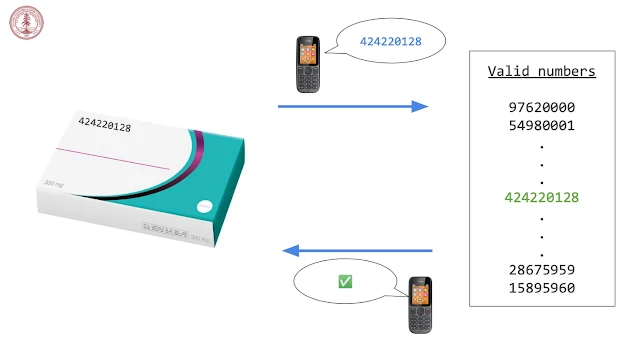# Stanford Python程式課 - 第四週

## 第四週學習目標Code in Place 第四週課程 Lesson 10: Lists

## Lesson 10: List 清單

### 學習重點

• 創建、修改清單 List
• 以迴圈方式存取清單元素 Loop over List

### Mutable vs Immutable when Passed as Parameters

• Mutable: variables act like they are copied
• Immutable: variables act lie their URL is copiedLesson 10 Lists: Mutable vs Immutable Variables

Lecture 10-1: Intro

Lecture 10-2: Using the console

Lecture 10-3: Lists

Lecture 10-4: List functions

Lecture 10-5: List looping

Lecture 10-6: List as params

Lecture 10-7: Average scores

## Lesson 11: Text 文字Code in Place 範例：mPedigree 公司利用字串簡訊解決偽藥問題

### 學習重點

• 了解Python的字串
• 字串是 immutable
• 以迴圈處理字串、創建新字串

Lecture 11-1: Introduction

Lecture 11-2: Revisiting Strings

Lecture 11-3: Immutability

Lecture 11-4: String Functions

Lecture 11-5: String Processing

Lecture 11-6: mPedigree Goldenkeys

### List 程式範例

listexamples.py

```"""
File: listexamples.py
---------------------
This program contains examples of using different functionality of
lists to show how you can do different list operations.
"""

def access_list_elements():
"""
Shows an example of accessing individual elements in a list
as well as overwriting a value.
"""
num_list = [10, 20, 30, 40, 50]
print('num_list =', num_list)
print('num_list =', num_list)
print('num_list =', num_list)
print('Setting num_list to 15')
num_list = 15
print('num_list =', num_list)

def print_individual_list_elements():
"""
Shows an example of using a for-loop with range to iterate
through the elements of a list.
"""
letters = ['a', 'b', 'c', 'd', 'e']
print('letters =', letters)
print('length of letters =', len(letters))
for i in range(len(letters)):
print(i, "->", letters[i])

def appending_elements():
"""
Shows two examples of using appending elements onto lists.
"""
alist = [10, 20, 30]
print('alist =', alist)
print('Appending 40 to alist')
alist.append(40)
print('Appending 50 to alist')
alist.append(50)
print('alist =', alist)

new_list = []
print('new_list =', new_list)
print('Appending a to new_list')
new_list.append('a')
print('Appending 4.3 to new_list')
new_list.append(4.3)
print('new_list =', new_list)

def popping_elements():
"""
Shows an example of popping elements off a list.
"""
alist = [10, 20, 30, 40, 50]
print('alist =', alist)
x = alist.pop()
print('popped element from alist, got', x)
print('alist =', alist)
x = alist.pop()
print('popped element from alist, got', x)
print('alist =', alist)
x = alist.pop()
print('popped element from alist, got', x)
print('alist =', alist)
x = alist.pop()
print('popped element from alist, got', x)
print('alist =', alist)
x = alist.pop()
print('popped element from alist, got', x)
print('alist =', alist)

def look_up_elements():
"""
Shows an example of using 'in' to check if a value is contained in
a list.
"""
str_list = ['Ruth', 'John', 'Sonia']
print('str_list =', str_list)
if 'Sonia' in str_list:
print('Sonia is in str_list')

def for_each_loop():
"""
Shows an example of using a for-each loop with a list.
"""
str_list = ['Ruth', 'John', 'Sonia']
for elem in str_list:
print(elem)

def main():
"""
We call a number of different functions that show the results
of different list operations to show examples of how they work.
"""
access_list_elements()
print_individual_list_elements()
appending_elements()
popping_elements()
look_up_elements()
for_each_loop()

if __name__ == '__main__':
main()
```

listparameter.py

```"""
File: listparameter.py
---------------------
This program contains examples of using lists as parameters,
especially in different type of for loops.
"""

"""
Attempts to add 5 to all the elements in the list.
BUGGY CODE!  Does not actually add 5 to the list elements
if values are primitive type (like int or float), since
value would just be a local copy of such variables.
"""
for value in alist:
value += 5

"""
Successfully adds 5 to all the elements in the list.
Changes made to the list persist after this function ends
since we are changing actual values in the list.
"""
for i in range(len(alist)):
alist[i] += 5

def create_new_list(alist):
"""
Shows an example of what happens when you create a new list
that has the same name as a parameter in a function.  After
you create the new list, you are no longer referring to the
parameter "alist" that was passed in
"""
# This "alist" is referring to the parameter "alist"
alist.append(9)

# Creating a new "alist". This is not the same as the parameter
# passed in.  After this line references to "alist" are no longer
# referring to the parameter "alist" that was passed in.
alist = [1, 2, 3]

def main():
values = [5, 6, 7, 8]
print('values =', values)

print('values =', values)

print('values =', values)

values = [5, 6, 7, 8]
create_new_list(values)
print('values =', values)

if __name__ == '__main__':
main()
```

averagescores.py

```"""
File: averagescores.py
----------------------
This program reads score values from a user and computes their
average.  It also adds extra credit to all the scores and computes
the new average.
"""

EXTRA_CREDIT = 5

def get_scores():
"""
Asks the user for a list of scores (numbers).
Returns a list containing the scores
"""
score_list = []
while True:
score = float(input("Enter score (0 to stop): "))
if score == 0:
break
score_list.append(score)
return score_list

def compute_average(score_list):
"""
Returns the average value of the list of scores passed in.
"""
num_scores = len(score_list)
total = sum(score_list)

def give_extra_credit(score_list, extra_credit_value):
"""
Adds extra_credit_value to all the values in score_list.
"""
for i in range(len(score_list)):
score_list[i] += extra_credit_value

def print_list(alist):
"""
Prints all the values in the list passed in
"""
for value in alist:
print(value)

def main():
"""
Computes average for a set of scores entered by the user.
Then adds extra credit to scores and recomputes average.
"""
scores = get_scores()
print("Scores are:")
print_list(scores)
avg = compute_average(scores)
print('Average score is', avg)

give_extra_credit(scores, EXTRA_CREDIT)
print("New scores are:")
print_list(scores)
avg = compute_average(scores)
print('New average is', avg)

if __name__ == '__main__':
main()
```

### Text 程式範例

goldkeys.py:

```import random

N_LABELS = 5000

def main():
for i in range(N_LABELS):
id = rand_part + unique_part
print(id)

"""
'0042'
'100'
"""
num_string = str(num)
while len(num_string) < length:
# insert a 0 at the start of the string, increasing its length by one
num_string = "0" + num_string
return num_string

if __name__ == "__main__":
main()
```